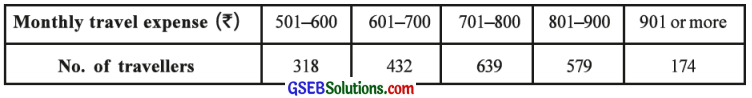# GSEB Solutions Class 12 Statistics Part 2 Chapter 1 Probability Ex 1.5

Gujarat Board Statistics Class 12 GSEB Solutions Part 2 Chapter 1 Probability Ex 1.5 Textbook Exercise Questions and Answers.

## Gujarat Board Textbook Solutions Class 12 Statistics Part 2 Chapter 1 Probability Ex 1.5

Question 1.
The sample data about monthly travel expense (in?) of a large group of travellers of local bus in a megacity are given in the following table:One person from this megacity travelling by local bus is randomly selected. Find the probability that the monthly travel expense of this person will be
(1) more than ₹ 900
(2) at the most ₹ 700
(3) ₹ 601 or more but ₹ 900 or less.
Here, the number of travellers selected in the sample is n = 318 + 432 + 639 + 579 + 174 = 2142

(1) A = Event that the monthly travel expense of the persons is more than ₹ 900.
∴ P(A) = Relative frequency of the number of travellers whose monthly travel expense is more than ₹ 900
= $$\frac{\text { No. of travellers whose monthly travel expense is more than } ₹ 900}{\text { Total no. of travellers }}$$
= $$\frac{m}{n}$$ = $$\frac{174}{2142}$$ = $$\frac{29}{357}$$(2) B = Event that the monthly travel expense of the persons is at the most ₹ 700.
∴ P(B) = Relative frequency of the number of travellers whose monthly travel expense is at the most ₹ 700
= $$\frac{\text { No. of travellers whose monthly travel expense is at the most } ₹ 700}{\text { Total no. of travellers }}$$
= $$\frac{m}{n}$$ = $$\frac{318+432}{2142}$$ = $$\frac{750}{2142}$$ = $$\frac{125}{357}$$

(3) C = Event that the monthly travel expense of the persons is ₹ 601 or more but ₹ 900 or less.
∴ P (C) = Relative frequency of the number of travellers whose monthly travel expense is ₹ 601 or more but ₹ 900 or less
= $$\frac{\text { No. of travellers whose monthly travel expense is } ₹ 601 \text { or more but } ₹ 900 \text { or less }}{\text { Total no. of travellers }}$$
= $$\frac{m}{n}$$ = $$\frac{432+639+579}{2142}$$ = $$\frac{1650}{2142}$$ = $$\frac{275}{357}$$

Question 2.
The details of a sample inquiry of 4979 voters of constituency are as follows:

 Year Time t ŷ = 177.8 + 5.6t 2011 1 177.8 + 5.6 (1) = 183.4 2012 2 177.8 + 5.6(2) = 189.0 2013 3 177.8 + 5.6 (3) = 194.6 2014 4 177.8 + 5.6 (4) = 200.2 2015 5 177.8 + 5.6 (5) = 205.8

One voter is randomly selected from this constituency.
(1) If this voter is a male, find the probability that he is a supporter of party A.
(2) If this voter is a supporter of party A, find the probability that he is a male.
Here, the total numbers of voters is n = 4979
A = Event that the selected voter is a male.
∴ P(A) = Relative frequency of male voters
= $$\frac{\text { No. of male voters }}{\text { Total no. of voters }}$$
= $$\frac{m}{n}$$

Putting, m = 1319 + 1217 = 2536 and n = 4979
P(A) = $$\frac{2536}{4979}$$
B = Event that the selected voter is supporter of party A.∴ P(B) = Relative frequency of voters who are supporters of party A
= $$\frac{\text { No. of voters who are supporters of party A }}{\text { Total no. of voters }}$$
= $$\frac{2437}{4979}$$

Putting, m = 1319 + 1118 = 2437 and n = 4979
P(B) = $$\frac{2437}{4979}$$
A ∩ B = Event that the selected voter is a male and supporter of party A .
∴ P(A ∩ B) = Relative frequency of the event A ∩ B
= \begin{aligned} &\text { No. of voters favourable for }\\ &=\frac{\text { the event } A \cap B}{\text { Total no. of voters }} \end{aligned}
= $$\frac{m}{n}$$
= $$\frac{1319}{4979}$$

(1) B|A = Event that the voter is a male that he is a supporter of party A
∴ P(B|A) = $$\frac{P(A \cap B)}{P(A)}$$
= $$\frac{\frac{1319}{4979}}{\frac{2536}{4979}}$$
= $$\frac{1319}{2536}$$

(2) A|B = Event that the voter is a supporter of party A that he is a male.
∴ P(A|B) = $$\frac{P(A \cap B)}{P(A)}$$
= $$\frac{\frac{1319}{4979}}{\frac{2437}{4979}}$$
= $$\frac{1319}{2437}$$